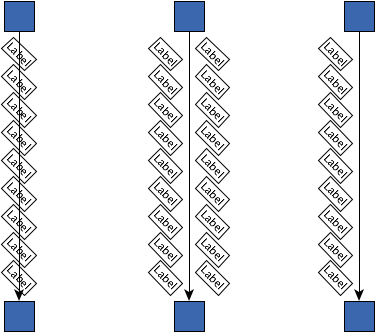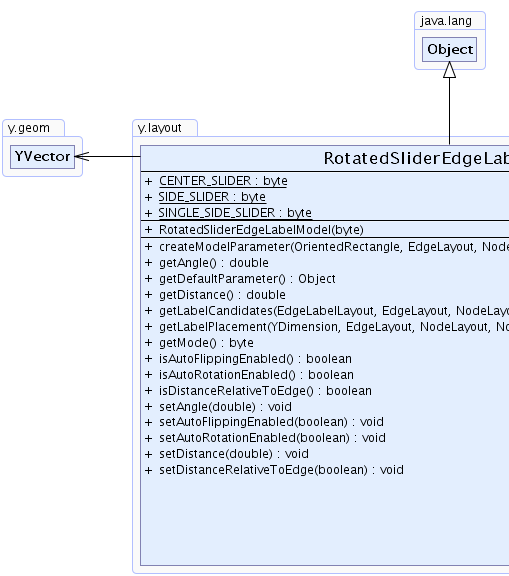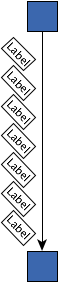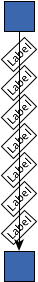Search this API

## y.layout Class RotatedSliderEdgeLabelModel

```java.lang.Objecty.layout.RotatedSliderEdgeLabelModel
```
All Implemented Interfaces:
EdgeLabelModel

`public class RotatedSliderEdgeLabelModelextends java.lang.Objectimplements EdgeLabelModel`

`RotatedSliderEdgeLabelModel` allows placement of labels at a set of continuous rotated positions along both sides of an edge or directly on the edge path.

It is possible to specify distance values that control the distance between label and edge and between label and nodes.`CENTER_SLIDER` mode on the left, `SIDE_SLIDER` mode in the middle and `SINGLE_SIDE_SLIDER` on the rightField Summary
`static byte` `CENTER_SLIDER`
Slider mode specifier which describes continuous label positions directly on the edge path.
`static byte` `SIDE_SLIDER`
Slider mode specifier which describes continuous label positions along the sides of the edge path.
`static byte` `SINGLE_SIDE_SLIDER`
Slider mode specifier which describes continuous label positions along one side of the edge path.

Constructor Summary
`RotatedSliderEdgeLabelModel(byte mode)`
Creates a new instance of `RotatedSliderEdgeLabelModel` with the given mode.

Method Summary
` java.lang.Object` ```createModelParameter(OrientedRectangle labelBounds, EdgeLayout edgeLayout, NodeLayout sourceNode, NodeLayout targetNode)```
Creates a model parameter that represents the given edge label position within this model.
` double` `getAngle()`
Returns the angle (measured in radians) of the label model.
` java.lang.Object` `getDefaultParameter()`
Returns a model parameter that encodes the default position of this model's allowed edge label positions.
` double` `getDistance()`
Returns the distance between the label's box and the edge's path.
` YList` ```getLabelCandidates(EdgeLabelLayout labelLayout, EdgeLayout edgeLayout, NodeLayout sourceLayout, NodeLayout targetLayout)```
Returns all `EdgeLabelCandidate`s that describe valid label positions within this model.
` OrientedRectangle` ```getLabelPlacement(YDimension labelSize, EdgeLayout edgeLayout, NodeLayout sourceNode, NodeLayout targetNode, java.lang.Object para)```
Returns the oriented box of the label for the position encoded by the given model parameter.
` byte` `getMode()`
Returns the model's slider mode which determines whether the label slides `on the edge`, `along both sides of the edge` or `along one side of the edge`.
` boolean` `isAutoFlippingEnabled()`
Returns whether or not edge labels get flipped if they would be upside down in their current position.
` boolean` `isAutoRotationEnabled()`
Returns whether or not edge labels are automatically rotated according to the angle of the corresponding reference edge segment.
` boolean` `isDistanceRelativeToEdge()`
Returns whether or not the distance to the edge is interpreted relative to the edge's path.
` void` `setAngle(double angle)`
Specifies the angle (measured in radians) of the label model.
` void` `setAutoFlippingEnabled(boolean autoFlippingEnabled)`
Specifies whether or not edge labels get flipped if they would be upside down in their current position.
` void` `setAutoRotationEnabled(boolean enabled)`
Specifies whether or not edge labels are automatically rotated according to the angle of the corresponding reference edge segment.
` void` `setDistance(double distance)`
Specifies the distance between the label's box and the edge's path.
` void` `setDistanceRelativeToEdge(boolean distanceRelativeToEdge)`
Specifies whether or not the distance to the edge is interpreted relative to the edge's path.

Methods inherited from class java.lang.Object
`clone, equals, finalize, getClass, hashCode, notify, notifyAll, toString, wait, wait, wait`

Field Detail

### CENTER_SLIDER

`public static final byte CENTER_SLIDER`
Slider mode specifier which describes continuous label positions directly on the edge path.

`getMode()`, Constant Field Values
Sample Graph:Labels are placed centered on the edge

### SIDE_SLIDER

`public static final byte SIDE_SLIDER`
Slider mode specifier which describes continuous label positions along the sides of the edge path.

`getMode()`, Constant Field Values
Sample Graph:Labels are placed beside the edge

### SINGLE_SIDE_SLIDER

`public static final byte SINGLE_SIDE_SLIDER`
Slider mode specifier which describes continuous label positions along one side of the edge path.

The side of the slider is determined by the `distance`. A positive distance will result in a right slider while negative distance will result in a left slider. Those sides are defined relative to the segment's direction.
`getMode()`, Constant Field Values
Sample Graph:Labels are placed at one side of the edge
Constructor Detail

### RotatedSliderEdgeLabelModel

`public RotatedSliderEdgeLabelModel(byte mode)`
Creates a new instance of `RotatedSliderEdgeLabelModel` with the given mode.

Parameters:
`mode` - the slider mode
`getMode()`
Method Detail

### getMode

`public byte getMode()`
Returns the model's slider mode which determines whether the label slides `on the edge`, `along both sides of the edge` or `along one side of the edge`.

Returns:
the model's slider mode

### isDistanceRelativeToEdge

`public boolean isDistanceRelativeToEdge()`
Returns whether or not the distance to the edge is interpreted relative to the edge's path.

If enabled, the label is placed to the left of the edge segment (relative to the segment direction) if the given distance is less than `0` and to the right of the edge segment if the given distance is greater than `0`.

If disabled, the label is placed below the edge segment (in geometric sense) if the distance is less than `0` and above the edge segment if the distance is greater than `0`.

Returns:
`true` if the distance is described relative to the edge, `false` otherwise
`setDistanceRelativeToEdge(boolean)`, `setDistance(double)`

### setDistanceRelativeToEdge

`public void setDistanceRelativeToEdge(boolean distanceRelativeToEdge)`
Specifies whether or not the distance to the edge is interpreted relative to the edge's path.

If enabled, the label is placed to the left of the edge segment (relative to the segment direction) if the given distance is less than `0` and to the right of the edge segment if the given distance is greater than `0`.

If disabled, the label is placed below the edge segment (in geometric sense) if the distance is less than `0` and above the edge segment if the distance is greater than `0`.

Default Value:
The default value is true. The distance to the edge is interpreted relative to the edge's path.
Parameters:
`distanceRelativeToEdge` - `true` if the distance should be described relative to the edge, `false` otherwise
`setDistance(double)`

### getDistance

`public double getDistance()`
Returns the distance between the label's box and the edge's path.

The interpretation of positive/negative values depends on property `isDistanceRelativeToEdge()`.
Returns:
the distance between the label's box and the edge's path
`setDistanceRelativeToEdge(boolean)`, `setDistance(double)`

### setDistance

`public void setDistance(double distance)`
Specifies the distance between the label's box and the edge's path.

The interpretation of positive/negative values depends on property `isDistanceRelativeToEdge()`.
Default Value:
The default value is 5.
Parameters:
`distance` - the distance between the label's box and the edge's path
`setDistanceRelativeToEdge(boolean)`
Sample Graphs:`5``20`

### isAutoRotationEnabled

`public boolean isAutoRotationEnabled()`
Returns whether or not edge labels are automatically rotated according to the angle of the corresponding reference edge segment.

Returns:
`true` if edge labels are automatically rotated according to the angle of the corresponding reference edge segment, `false` otherwise
`setAutoRotationEnabled(boolean)`

### setAutoRotationEnabled

`public void setAutoRotationEnabled(boolean enabled)`
Specifies whether or not edge labels are automatically rotated according to the angle of the corresponding reference edge segment.

Default Value:
The default value is true. Labels are rotated with their edge segment.
Parameters:
`enabled` - `true` if edge labels should be automatically rotated according to the angle of the corresponding reference edge segment, `false` otherwise
Sample Graphs:`false``true`

### isAutoFlippingEnabled

`public boolean isAutoFlippingEnabled()`
Returns whether or not edge labels get flipped if they would be upside down in their current position.

Returns:
`true` if edge labels get flipped if they would be upside down in their current position, `false` otherwise
`setAutoFlippingEnabled(boolean)`

### setAutoFlippingEnabled

`public void setAutoFlippingEnabled(boolean autoFlippingEnabled)`
Specifies whether or not edge labels get flipped if they would be upside down in their current position.

Default Value:
The default value is false. Edge labels keep their orientation.
Parameters:
`autoFlippingEnabled` - `true` if edge labels should get flipped if they would be upside down in their current position, `false` otherwise
Sample Graphs:`false``true`

### getAngle

`public double getAngle()`
Returns the angle (measured in radians) of the label model. The angle is applied in clockwise direction.

Returns:
the angle of the label model
`setAngle(double)`

### setAngle

`public void setAngle(double angle)`
Specifies the angle (measured in radians) of the label model. The angle is applied in clockwise direction.

Default Value:
The default value is 0.
Parameters:
`angle` - the angle of the label model
Sample Graphs:`0``-0.79`

### getDefaultParameter

`public java.lang.Object getDefaultParameter()`
Returns a model parameter that encodes the default position of this model's allowed edge label positions.

This parameter can be passed to `getLabelPlacement(YDimension, EdgeLayout, NodeLayout, NodeLayout, Object)` to retrieve the corresponding label box.

The default positions for the `slider modes` are relative to the first edge segment, either at the beginning of the segment (`SIDE_SLIDER` or `SINGLE_SIDE_SLIDER`) or at its middle (`CENTER_SLIDER`).

Specified by:
`getDefaultParameter` in interface `EdgeLabelModel`
Returns:
the model parameter describing the default placement of the edge label
`getMode()`

### getLabelPlacement

```public OrientedRectangle getLabelPlacement(YDimension labelSize,
EdgeLayout edgeLayout,
NodeLayout sourceNode,
NodeLayout targetNode,
java.lang.Object para)```
Description copied from interface: `EdgeLabelModel`
Returns the oriented box of the label for the position encoded by the given model parameter.

Specified by:
`getLabelPlacement` in interface `EdgeLabelModel`
Parameters:
`labelSize` - the width and height of the label
`edgeLayout` - the layout of the edge to which the label belongs
`sourceNode` - the layout of the source node of the label-owning edge
`targetNode` - the layout of the target node of the label-owning edge
`para` - the model parameter that describes the abstract position of the label within this model
Returns:
the oriented bounds of the label

### getLabelCandidates

```public YList getLabelCandidates(EdgeLabelLayout labelLayout,
EdgeLayout edgeLayout,
NodeLayout sourceLayout,
NodeLayout targetLayout)```
Returns all `EdgeLabelCandidate`s that describe valid label positions within this model. The number of candidates and their respective locations are computed depending on the geometries of both label and edge.

Specified by:
`getLabelCandidates` in interface `EdgeLabelModel`
Parameters:
`labelLayout` - the label for which candidates should be generated
`edgeLayout` - the layout of the edge to which the label belongs
`sourceLayout` - the layout of the source node of the label-owning edge
`targetLayout` - the layout of the target node of the label-owning edge
Returns:
a list of `EdgeLabelCandidate` instances

### createModelParameter

```public java.lang.Object createModelParameter(OrientedRectangle labelBounds,
EdgeLayout edgeLayout,
NodeLayout sourceNode,
NodeLayout targetNode)```
Description copied from interface: `EdgeLabelModel`
Creates a model parameter that represents the given edge label position within this model.

The created model parameter is the closest parameter representation of the given label location that can be achieved within this model.

This parameter can be passed to `EdgeLabelModel.getLabelPlacement(YDimension, EdgeLayout, NodeLayout, NodeLayout, Object)` to retrieve the current label box.

A model parameter can be an arbitrary `Object`. However it must contain all information to allow restoring of the encoded location with this `EdgeLabelModel`.

Specified by:
`createModelParameter` in interface `EdgeLabelModel`
Parameters:
`labelBounds` - the oriented box of the label, encoding the label location for which the parameter should be created
`edgeLayout` - the layout of the edge to which the label belongs
`sourceNode` - the layout of the source node of the label-owning edge
`targetNode` - the layout of the target node of the label-owning edge
Returns:
the model parameter representing the given label location
`EdgeLabelModel.getLabelPlacement(YDimension, EdgeLayout, NodeLayout, NodeLayout, Object)`